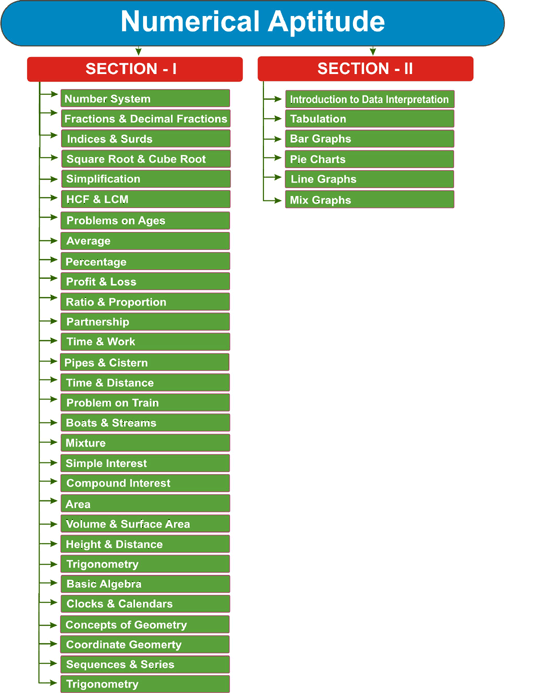NEW!# (Guidance Programme) Combined Graduate Level Examination - Tier - II: Paper - 1 : Arithmetic Ability

## Paper-I : Arithmetic Ability

The questions will be designed to test the ability of appropriate use of numbers and number sense of the candidate. The scope of the test will be the computation of whole numbers, decimals, fractions and relationships between numbers, Percentage. Ratio & Proportion, Square roots, Averages, Interest, Profit and Loss, Discount, Partnership Business, Mixture and Allegation, Time and distance, Time & Work, Basic algebraic identities of School Algebra & Elementary surds, Graphs of Linear Equations, Triangle and its various kinds of centres, Congruence and similarity of triangles, Circle and its chords, tangents, angles subtended by chords of a circle, common tangents to two or more circles, Triangle, Quadrilaterals, Regular Polygons , Circle, Right Prism, Right Circular Cone, Right Circular Cylinder, Sphere, Hemispheres, Rectangular Parallelepiped, Regular Right Pyramid with triangular or square base, Trigonometric ratio, Degree and Radian Measures, Standard Identities, Complementary angles, Heights and Distances, Histogram, Frequency polygon, Bar diagram & Pie chart.
There is one topics on Data Analysis too, Data Analysis is sum total of various operations pertaining to data.

An arrayed data is classified, grouped and useful conclusions are derived under the heading Data Analysis. The emphasis in this topic is on fundamental concepts which are most important part in Mathematics. You should remember all the formulae that might be very useful from examination point of view. But, as there is no end to variety of questions, the list of formulae will be endless. So, it is opportune understanding the basics more importantly than the formulae. Direct formulae or Quicker methods save your valuable time but they have very high potential of creating confusion in this usage. So you should know where the particular formula should be useful. A little change in the questions may lead you to wrong solution. So be careful before using them. It is a compulsory paper you can’t ignore or neglect. So, make sure you are ready to improve your mathematical skills. Each questions values 2 marks whereas each question of English values 1 marks in CGL tier two. So it is require you to devote relatively more time on this paper to get more marks. Also, answer of Maths questions are more confirmed than other sections like English, reasoning, which is often confusing. Most of us feel that it is time consuming but using short-cut method you will able to solve the questions in a fraction of minute. For this, need great amount of Practice. Read and solve Maths problems as much as you can. You are suggested to go through as many examples as possible.

Each questions given in examples has some uniqueness. Mark it and keep it in mind. To collect more examples of different types you may consult different books available in the market. You should have to cover all the chapters that are given in the syllabus. Also, you should know the pattern and style of the questions paper of the exam. If you can, you should arrange as many as possible numbers of previous and sample papers. There are many sources: Guides, Books, Magazines etc. Clear all basics of mathematics and remember table from 2-30, also remember square root and cube root upto 50. For CGL more emphasis should be on the topics like Average, Ratio & Proportion, Profit & Loss, Percentage, Time & Work, Train, Speed Mensuration, etc. Here are some suggested reading; Quantitative Aptitude by R.S. Aggarwal, Magical Book on Quicker Maths by M. Tyra.